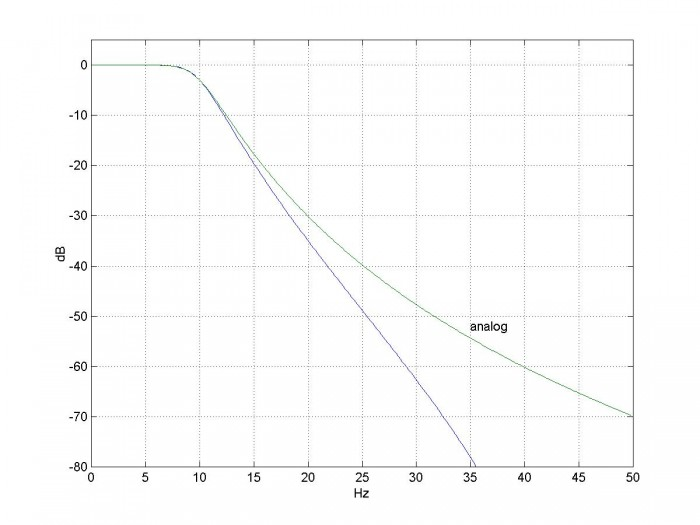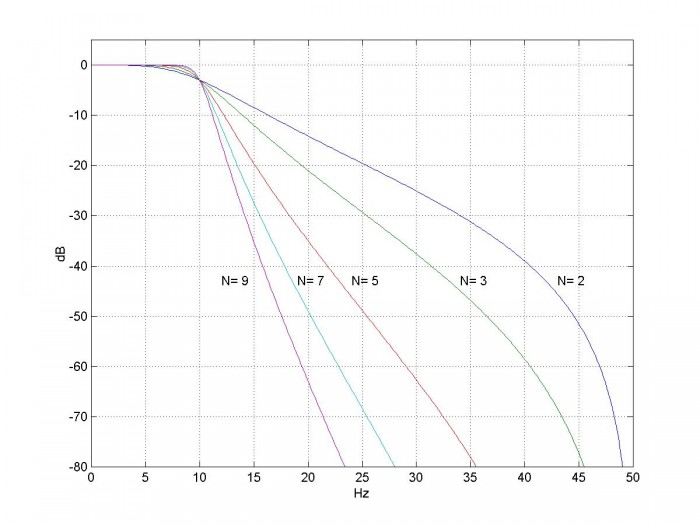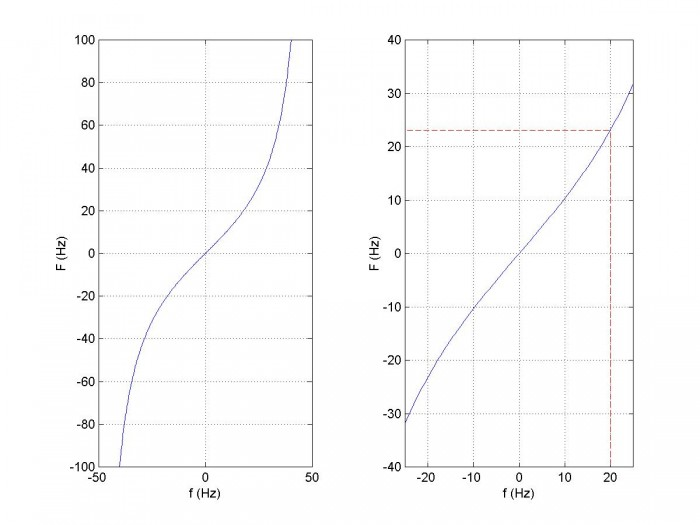# Design IIR Butterworth Filters Using 12 Lines of Code

While there are plenty of canned functions to design Butterworth IIR filters , it’s instructive and not that complicated to design them from scratch.  You can do it in 12 lines of Matlab code.  In this article, we’ll create a Matlab function butter_synth.m to design lowpass Butterworth filters of any order.  Here is an example function call for a 5th order filter:

N= 5           % Filter order
fc= 10;        % Hz cutoff freq
fs= 100;       % Hz sample freq
[b,a]= butter_synth(N,fc,fs)
b =    0.0013    0.0064    0.0128    0.0128    0.0064    0.0013
a =    1.0000   -2.9754    3.8060   -2.5453    0.8811   -0.1254


Then, to find the frequency response:

[h,f]= freqz(b,a,256,fs);
H= 20*log10(abs(h));


The magnitude response of the 5th order filter is shown in Figure 1, along with the response of the analog prototype.Figure 1.  Magnitude response of N= 5 IIR Butterworth filter with fc = 10 Hz and fs = 100 Hz.  The prototype analog filter’s response is also shown.

## Notation

First, a word about notation.  We need to distinguish frequency variables in the continuous-time (analog) world from those in the discrete-time world.  In this article, the following notation for frequency will be used:

continuous frequency                                     F  Hz

complex frequency                                          s = σ + jΩ

discrete frequency                                           f  Hz

discrete normalized radian frequency            ω = 2πf/fsradians, where fs = sample freq

## Background

Analog Butterworth filters have all-pole transfer functions.  For example, a third-order Butterworth filter with Ωc = 1 rad/s has the transfer function:

$$H(s)=\frac{1}{s^3+2s^2+2s+1}$$

or

$$H(s)=\frac{1}{(s-p_{a0})(s-p_{a1})(s-p_{a2}) }\qquad(1)$$

where the subscript a denotes analog (s-plane) poles.  The poles in the s-plane are:

pa0 =  -.5 + j.866

pa1 =   -1

pa2 =   -.5 -j.866

We will transform the poles in the s-plane to poles in the z-plane using the bilinear transform [2,3].  The bilinear transform converts H(s) to H(z) by replacing s with:

$$s=2f_s\frac{1-z^{-1}}{1+z^{-1}}\qquad(2)$$

where fs is sample frequency.  If we solve for z, we get:

$$z=\frac{1+s/(2f_s)}{1-s/(2f_s)}\qquad(3)$$

Equation 3 maps a point on the s plane to a point on the z plane.  For example, if fs= 2 Hz, the s-plane real pole at -1 maps to:

$$p=\frac{1-1/4}{1+1/4}=0.6$$

For the 3rd order filter, with Ωc=  1 and fs= 2, the z-plane poles fall as shown in Figure 2.

From equation 1, H(s) has 3 zeros at s= ∞.  How do they map to the z plane?  We will show later that the bilinear transform maps -∞ to ∞ on the jΩ axis  to -fs/2 to fs/2 on the unit circle.  So the 3 zeros of H(s) map to +/- fs/2 on the unit circle, which corresponds to z= -1.  (Recall that on the unit circle, z= e, where ω = 2πf/fs.  For f = +/-fs/2, we have ω = +/-π, so z = e = -1).  The three zeros are represented by the ‘o’ in figure 2.

We can now write the 3rd-order Butterworth H(z) as:

$$H(z)=K\frac{(z+1)(z+1)(z+1)}{(z-p_0)(z-p_1)(z-p_2)}\qquad(4)$$

where, from equation 3, p= [0.7143 +j 0.33   0.6   0.7143 -j 0.33].  Expanding the numerator and denominator, we have:

$$H(z)=K\frac{b_0z^3+b_1z^2+b_2z+b_3}{z^3+a_1z^2+a_2z+a_3}$$

$$H(z)=K\frac{b_0+b_1z^{-1}+b_2z^{-2}+b_3z^{-3}}{1+a_1z^{-1}+a_2z^{-2}+a_3z^{-3}}\qquad(5)$$

Where b = [1 3 3 1] and a= [1 -2.0286 1.4762 -0.3714].  K is chosen to make gain = 1 at ω= 0:  K = 1/H(ω=0) = 1/H(z=1) = sum(a)/sum(b)= .00952

Looking again at Figure 1, you may have wondered why the attenuation of the IIR filter is greater than that of the analog filter as f approaches fs/2.  The reason is that the analog filter’s zeros are at ∞, while the bilinear transform compresses the frequency scale so that the IIR filter’s zeros are at fs/2.Figure 2.  Z-plane Poles and zeros of 3rd order IIR Butterworth filter with Ωc=  1 and fs= 2.

## Filter Synthesis

Here is a summary of the steps for finding the filter coefficients :

1. Find the poles of the analog prototype filter with Ωc = 1 rad/s.
2. Given the desired fc of the digital filter, find the corresponding analog frequency Fc.
3. Scale the s-plane poles by 2πFc.
4. Transform the poles from the s-plane to the z-plane.
5. Add N zeros at z = -1.
6. Convert poles and zeros to polynomials with coefficients an and bn.

Now let’s look at the steps in detail.  Note we’ll repeat a lot of the math we already presented above.  A Matlab function butter_synth that performs the filter synthesis is provided in the Appendix.  It gives the same results as the built-in Matlab function butter(n,Wn) .

1.  Poles of the analog filter.  For a Butterworth filter of order N with Ωc = 1 rad/s, the poles are given by [4,5]:

$$p_{ak}= -sin(\theta)+jcos(\theta)$$

where $$\theta=\frac{(2k-1)\pi}{2N},\quad k=1:N$$

2.  Given the desired fc, find analog frequency Fc.  As we’ll show in the next section, the bilinear transform does not map the analog frequency F to discrete frequency f linearly.  To achieve a digital filter cut-off frequency of fc, the analog prototype cut-off frequency must be:

$$F_c=\frac{f_s}{\pi}tan\left(\frac{\pi f_c}{f_s}\right)$$

This exercise is called frequency pre-warping.  For example, if fs= 100 Hz and we want fc= 20 Hz, then Fc = 23.13 Hz.

3.  Scale the s-plane poles by 2πFc. The poles obtained in step 1 gave Ωc = 1 rad/s (i.e. 1/(2π) Hz).  Multiplying the poles by 2πFc scales the analog filter cut-off frequency to Fc and the digital filter cut-off frequency to fc.

4.  Transform the poles from the s-plane to the z-plane using the bilinear transform.  From equation 3,

$$p_k=\frac{1+p_{ak}/(2f_s)}{1-p_{ak}/(2f_s)},\quad k=1:N$$

5.  Add N zeros at z = -1.  Following the example of equation 4, the numerator of H(z) is                (z + 1)N , meaning there are N poles at z = -1.  We now can write H(z) as:

$$H(z)=K\frac{(z+1)^N}{(z-p_0)(z-p_1)...(z-p_{N-1})}\qquad(6)$$

In butter_synth, we represent the N zeros as a vector q= -ones(1,N).

6.  Convert poles and zeros to polynomials with coefficients an and bn.  If we expand the numerator and denominator of equation 6, we get polynomials in z-n:

$$H(z)=K\frac{b_0+b_1z^{-1}+...+b_Nz^{-N}}{1+a_1z^{-1}+...+a_Nz^{-N}}\qquad(7)$$

The Matlab code to perform the expansion is:

a= poly(p)
a= real(a)
b= poly(q)


We want H(z) to have a gain of 1 at ω= 0.  Letting z= e, we have z= 1.  Then, referring to equation 7, we have gain at ω= 0 of:

$$H(z=1)=K\frac{\sum b}{\sum a}$$

So, for gain of 1 at ω= 0, we make $K=\sum a/\sum b$.

And that's the last step.  Figure 3 shows the frequency response vs. order N for filters synthesized by butter_synth.  Figure 4 shows the impulse response vs. order N for three cases.Figure 3.  IIR Butterworth magnitude responses for fc= 10 Hz and fs= 100 Hz.

[h,f]= freqz(b,a,256,fs);
H= 20*log10(abs(h));<\pre>Figure 4.  IIR Butterworth impulse responses for fc = 1 kHz and fs = 32 kHz.

x= [1 zeros(1,95)];  % impulse
y= filter(b,a,x);    % impulse response


## Frequency Mapping of the Bilinear Transform

The bilinear transform does not map the continuous frequency F to discrete frequency f linearly.   To show this, we evaluate equation 2 for s= jΩ and z= e:

$$j\Omega=2f_s\frac{1-e^{-j\omega}}{1+e^{-j\omega}}$$

$$j\Omega=j2f_stan(\omega/2)$$

Now substitute Ω= 2πF and ω= 2πf/fs:

$$F=\frac{f_s}{\pi}tan\left(\frac{\pi f}{f_s}\right)\qquad(8)$$

Figure 5 plots equation 8 for fs= 100 Hz.  The entire analog frequency range maps to  –fs/2 to fs/2.  Also shown on the zoomed plot on the right is the transformation of discrete frequency f = 20 Hz to continuous frequency F = 23.13 Hz.  Note that the frequency mapping is approximately linear for f < fs/10 or so.

Figure 6 shows the effect of using equation 8 to pre-warp the cut-off frequency of an analog prototype filter to give fc = 20 Hz.  With pre-warping, the analog prototype poles were scaled by 2π*23.13.  Without pre-warping, they were scaled by 2π*20.Figure 5.  Frequency mapping of the bilinear transform for fs = 100 Hz.  x axis is discrete frequency and y-axis is continuous frequency.  The right plot is a zoomed version of the left plot, showing the value of F for f = 20 Hz.Figure 6.  Effect of pre-warping for fc = 20 Hz and fs = 100 Hz.  5th order IIR Butterworth.

## References

1.  Mathworks website https://www.mathworks.com/help/signal/ref/butter.html

2.  Oppenheim, Alan V. and Shafer, Ronald W., Discrete-Time Signal Processing, Prentice Hall, 1989, section 7.1.2

3.  Lyons, Richard G., Understanding Digital Signal Processing, 2nd Ed., Pearson, 2004, section 6.5

4.  Williams, Arthur B. and Taylor, Fred J., Electronic Filter Design Handbook, 3rd Ed., McGraw-Hill, 1995, section 2.3

5.  Analog Devices Mini Tutorial MT-224, 2012 http://www.analog.com/media/en/training-seminars/tutorials/MT-224.pdf

Neil Robertson   December 2017

## Appendix    Matlab Function butter_synth  (12 lines of code, excluding error check)

This program is provided as-is without any guarantees or warranty.  The author is not responsible for any damage or losses of any kind caused by the use or misuse of the program.

% butter_synth.m    12/9/17 Neil Robertson
% Find the coefficients of an IIR butterworth lowpass filter
% using bilinear transform
%
% N= filter order
% fc= -3 dB frequency in Hz
% fs= sample frequency in Hz
% b = numerator coefficients of digital filter
% a = denominator coefficients of digital filter
%
function [b,a]= butter_synth(N,fc,fs);
%
if fc>=fs/2;
error('fc must be less than fs/2')
end
% I.  Find poles of analog filter
k= 1:N;
theta= (2*k -1)*pi/(2*N);
pa= -sin(theta) + j*cos(theta);     % poles of filter with cutoff = 1 rad/s
%
% II.  scale poles in frequency
Fc= fs/pi * tan(pi*fc/fs);          % continuous pre-warped frequency
pa= pa*2*pi*Fc;                     % scale poles by 2*pi*Fc
%
% III.  Find coeffs of digital filter
% poles and zeros in the z plane
p= (1 + pa/(2*fs))./(1 - pa/(2*fs));      % poles by bilinear transform
q= -ones(1,N);                   % zeros
%
% convert poles and zeros to polynomial coeffs
a= poly(p);                   % convert poles to polynomial coeffs a
a= real(a);
b= poly(q);                   % convert zeros to polynomial coeffs b
K= sum(a)/sum(b);             % amplitude scale factor
b= K*b;


Previous post by Neil Robertson:
Digital PLL's -- Part 2

[ - ]
Comment by December 19, 2017Thanks Neil,

You cleared up the internal secrets of Matlab function "butter" and I got identical results using either. Great job and very useful.

[ - ]
Comment by December 19, 2017Kaz,

Yes, I was pleasantly surprised when I ran the function and the results matched.  So now only another 99.9% of Matlab is a black box.

Neil

[ - ]
Comment by December 19, 2017Hi Sir,

Clarity in your articles while dealing with fundamental concepts is great.

Thank you,

Sreekanth

[ - ]
Comment by December 19, 2017Sreekanth,

You're welcome, I appreciate the feedback.

Neil

[ - ]
Comment by December 20, 2017I request you to do one article like this on iir notch filter (if you have time).

Sreekanth

[ - ]
Comment by December 20, 2017I'll put that on my list!

Neil

[ - ]
Comment by August 14, 2018Hey there,

When I started looking for an algorithm to design Butterworth filter I wanted to escape a somewhat bug or limitation from matlab keeping me from designing filter with superlow cutt-off frequency, for instance by running the following code:

butter(2,1e-9);

It seems going too low does not do well with Matlab, supprinsingly your code also does not work with such cut-off frequency, do you have any idea what might cause that?

Cheers and thanks again for your work

[ - ]
Comment by August 14, 2018Dermiste,

See my article on iir filter design using Cascaded biquads.  It explains the problem you are having.

Regards,

Neil

[ - ]
Comment by August 16, 2018All right it seems to be a solution although my filter is of order 2, and regardless the method (Biquad or Butter) I always end up having a gain K of 0 when summing my coefs "a". For the record I need a cut-off frequency at 2.365968869395443e-09.

I guess the improved narrowband you talk about in another article will do the trick? I am about to give it a try.

Thanks for you work !

[ - ]
Comment by August 16, 2018Dermiste,

That is a really low cut-off frequency.  What is your application?

Note that you could use a decimator (e.g. CIC decimator) to reduce your sample rate and make the problem more tractable.

regards,

Neil

[ - ]
Comment by August 16, 2018The application is DC value extraction with lowest noise possible. It is means to be implemented on GS/s ADC at a low power requirement.

Maybe I'm not approaching this the right way, I should filter smarter instead of harder (with butterworth 2nd order filter)

To post reply to a comment, click on the 'reply' button attached to each comment. To post a new comment (not a reply to a comment) check out the 'Write a Comment' tab at the top of the comments.